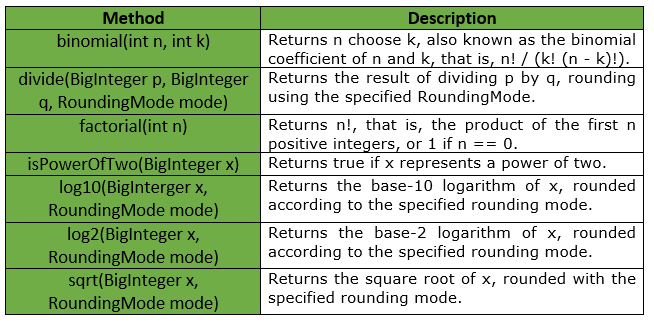# BigIntegerMath Class | Guava | Java

BigIntegerMath is used to perform mathematical operations on BigInteger values. Basic standalone math functions are divided into the classes IntMath, LongMath, DoubleMath, and BigIntegerMath based on the primary numeric type involved. These classes have parallel structure, but each supports only the relevant subset of functions. Similar functionality for int and for long can be found in IntMath and LongMath respectively.

Declaration : The declaration for com.google.common.math.BigIntegerMath class is :

```@GwtCompatible(emulated = true)
public final class BigIntegerMath
extends Object
```

Below table shows the methods provided by BigIntegerMath Class of Guava :Exceptions :

• log2 : IllegalArgumentException if x <= 0
• log10 : IllegalArgumentException if x <= 0
• sqrt : IllegalArgumentException if x < 0
• divide : ArithmeticException if q == 0, or if mode == UNNECESSARY and a is not an integer multiple of b
• factorial : IllegalArgumentException if n < 0
• binomial : IllegalArgumentException if n < 0, k n

Example 1 :

 `// Java code to show implementation of ` `// BigIntegerMath Class of Guava ` `import` `java.math.*; ` `import` `com.google.common.math.BigIntegerMath; ` ` `  `class` `GFG { ` ` `  `    ``// Driver code ` `    ``public` `static` `void` `main(String args[]) ` `    ``{ ` ` `  `        ``// Creating an object of GFG class ` `        ``GFG obj = ``new` `GFG(); ` ` `  `        ``// Function calling ` `        ``obj.examples(); ` `    ``} ` ` `  `    ``private` `void` `examples() ` `    ``{ ` ` `  `        ``try` `{ ` ` `  `            ``// exception will be thrown as 10 is ` `            ``// not completely divisible by 3 ` `            ``// thus rounding is required, and ` `            ``// RoundingMode is set as UNNESSARY ` `            ``System.out.println(BigIntegerMath.divide(BigInteger.TEN, ` `                                                ``new` `BigInteger(``"3"``),  ` `                                           ``RoundingMode.UNNECESSARY)); ` `        ``} ` `        ``catch` `(ArithmeticException ex) { ` `            ``System.out.println(``"Error Message is : "` `+ ` `                                       ``ex.getMessage()); ` `        ``} ` `    ``} ` `} `

Output:

```Error Message is : Rounding necessary
```

Example 2 :

 `// Java code to show implementation of ` `// BigIntegerMath Class of Guava ` `import` `java.math.*; ` `import` `com.google.common.math.BigIntegerMath; ` ` `  `class` `GFG { ` ` `  `    ``// Driver code ` `    ``public` `static` `void` `main(String args[]) ` `    ``{ ` ` `  `        ``// Creating an object of GFG class ` `        ``GFG obj = ``new` `GFG(); ` ` `  `        ``// Function calling ` `        ``obj.examples(); ` `    ``} ` ` `  `    ``private` `void` `examples() ` `    ``{ ` ` `  `        ``// As 10 is divisible by 5, so ` `        ``// no exception is thrown ` `        ``System.out.println(BigIntegerMath.divide(BigInteger.TEN, ` `                                        ``new` `BigInteger(``"5"``), ` `                                    ``RoundingMode.UNNECESSARY)); ` ` `  `        ``// To compute log to base 10 ` `        ``System.out.println(``"Log10 is : "` `+  ` `             ``BigIntegerMath.log10(``new` `BigInteger(``"1000"``),  ` `                                 ``RoundingMode.HALF_EVEN)); ` ` `  `        ``// To compute factorial ` `        ``System.out.println(``"factorial is : "` `+  ` `                         ``BigIntegerMath.factorial(``7``)); ` ` `  `        ``// To compute log to base 2 ` `        ``System.out.println(``"Log2 is : "` `+  ` `           ``BigIntegerMath.log2(``new` `BigInteger(``"8"``), ` `                          ``RoundingMode.HALF_EVEN)); ` ` `  `        ``// To compute square root ` `        ``System.out.println(``"sqrt is : "` `+        ` `                    ``BigIntegerMath.sqrt(BigInteger. ` `                    ``TEN.multiply(BigInteger.TEN), ` `                        ``RoundingMode.HALF_EVEN)); ` `    ``} ` `} `

Output:

```2
Log10 is : 3
factorial is : 5040
Log2 is : 3
sqrt is : 10
```

Attention reader! Don’t stop learning now. Get hold of all the important Java and Collections concepts with the Fundamentals of Java and Java Collections Course at a student-friendly price and become industry ready.

My Personal Notes arrow_drop_upIn love with a semicolon because sometimes i miss it so badly)

If you like GeeksforGeeks and would like to contribute, you can also write an article using contribute.geeksforgeeks.org or mail your article to contribute@geeksforgeeks.org. See your article appearing on the GeeksforGeeks main page and help other Geeks.

Please Improve this article if you find anything incorrect by clicking on the "Improve Article" button below.

Article Tags :
Practice Tags :

Be the First to upvote.

Please write to us at contribute@geeksforgeeks.org to report any issue with the above content.